International
Tables for
Crystallography
Volume D
Physical properties of crystals
Edited by A. Authier

International Tables for Crystallography (2006). Vol. D, ch. 1.1, pp. 6-7

## Section 1.1.2.4. Covariant coordinates – dual or reciprocal space

A. Authiera*

aInstitut de Minéralogie et de la Physique des Milieux Condensés, Bâtiment 7, 140 rue de Lourmel, 75015 Paris, France
Correspondence e-mail: aauthier@wanadoo.fr

| top | pdf |

#### 1.1.2.4.1. Covariant coordinates

| top | pdf |

Using the developments (1.1.2.1)and (1.1.2.5), the scalar products of a vector x and of the basis vectorscan be written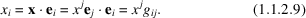The n quantitiesare called covariant components, and we shall see the reason for this a little later. The relations (1.1.2.9)can be considered as a system of equations of which the components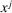are the unknowns. One can solve it since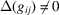(see the end of Section 1.1.2.2). It follows that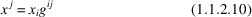with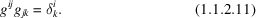The table of the's is the inverse of the table of the's. Let us now take up the development of x with respect to the basis: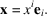Let us replaceby the expression (1.1.2.10):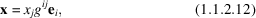and let us introduce the set of n vectors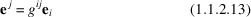which span the space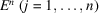. This set of n vectors forms a basis since (1.1.2.12)can be written with the aid of (1.1.2.13)as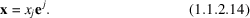The's are the components of x in the basis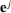. This basis is called the dual basis. By using (1.1.2.11)and (1.1.2.13), one can show in the same way that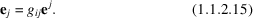It can be shown that the basis vectorstransform in a change of basis like the componentsof the physical space. They are therefore contravariant. In a similar way, the componentsof a vector x with respect to the basistransform in a change of basis like the basis vectors in direct space,; they are therefore covariant: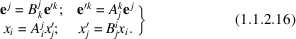#### 1.1.2.4.2. Reciprocal space

| top | pdf |

Let us take the scalar products of a covariant vector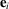and a contravariant vector: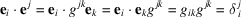[using expressions (1.1.2.5), (1.1.2.11)and (1.1.2.13)].

The relation we obtain,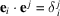, is identical to the relations defining the reciprocal lattice in crystallography; the reciprocal basis then is identical to the dual basis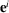.

#### 1.1.2.4.3. Properties of the metric tensor

| top | pdf |

In a change of basis, following (1.1.2.3)and (1.1.2.5), the's transform according to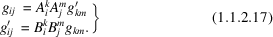Let us now consider the scalar products,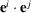, of two contravariant basis vectors. Using (1.1.2.11)and (1.1.2.13), it can be shown that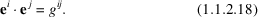In a change of basis, following (1.1.2.16), the's transform according to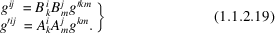The volumes V ′ and V of the cells built on the basis vectors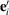and, respectively, are given by the triple scalar products of these two sets of basis vectors and are related by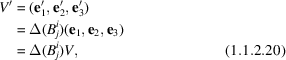where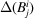is the determinant associated with the transformation matrix between the two bases. From (1.1.2.17)and (1.1.2.20), we can write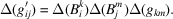If the basisis orthonormal,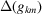and V are equal to one,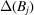is equal to the volume V ′ of the cell built on the basis vectorsand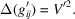This relation is actually general and one can remove the prime index: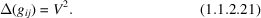In the same way, we have for the corresponding reciprocal basis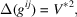whereis the volume of the reciprocal cell. Since the tables of the's and of the's are inverse, so are their determinants, and therefore the volumes of the unit cells of the direct and reciprocal spaces are also inverse, which is a very well known result in crystallography.